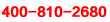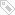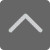# 一年级上册数学期末试卷-小学数学一年级上册第五单元测试题

2019-01-09 22:54:30 　来源：网络整理

一年级上册数学期末试卷-小学数学一年级上册第五单元测试题一年级的你是不是有在学校乖乖听老师的话？下面就是小编为大家带来的一年级上册数学期末试卷-小学数学一年级上册第五单元测试题，希望可以帮助到大家。

一、口算(20分)

3+6= 4+4= 9-6= 5+2= 8-6=

10-5= 9+1= 4+5= 7+3= 7-7=

6+2= 7-4= 10-7= 5+4= 8-0=

8-4= 5+5= 1+8= 4+3= 9-4=

二、填写(30分)

(1) 1

(4)6和8的中间的一个数是( )

(5)与6相邻的两个数是( )和( )

(6)比10少1的数是( )，比5多4的数是( )

(7)4前面的一个数是( )，后面的一个数是( )

(8)在6，0，9，5，7，1，4中把小于7的数写在下面的格子里

(9)最大的一位数是( )

三、在( )里填上合适的数(9分)

( )+2=6 7+( )=10 ( )-2=5

( )-9=1 5+( )=8 ( )+3=9

( )-3=4 9-( )=2 ( )-6=4

四、从4，5，8，9中选出3个数，写出两个加法算式和两个减法算式(4分)

五、连一连(6分)

3 + 4 10 3 + 2 + 4 5

9 - 5 7 9 –7 + 3 9

8 + 2 4 10 – 4 + 2 8

六、在○里填上>、<或 =(8分)

5+2 8 7-4 1 8 4+3 10-2 9

10-5 1+4 9+1 10-0 6+2 9-2 8+1 4+6

七、在○里填上 + 或 - (8分)

5 5=10 4 3=7 9 3=6 7 2=5

8 4=4 10 6=4 5 4=9 6 2=8

八、看图写算式(6分)

九、按要求完成下面各题(9分)

7 0 5 10 9 1

(1)上面一共有( )个数

(2)最大的数是( )，最小的数是( )

(3)5是在左数第( )个，从右数第5个数是( )

(4)7和10的中间有( )个数

(5)把左边3个数圈起来。

(6)把上面的数按顺序排一排。

( )>( )>( )>( )>( )>( )

小编推荐：

以上就是小编特意为大家整理的一年级上册数学期末试卷-小学数学一年级上册第五单元测试题希望对有需要的同学提供帮助，大家如果在学习中有什么疑问，欢迎拨打爱智康免费电话：!那里有专业的老师为大家解答。

### 2019年精品学习包：

•### 小学语|数|英

历年试卷、北京30所校练习题、名著|重点校巡礼|等100讲视频、纸质书籍领取

•### 初中九大学科

历年真题、期末|期中|志愿填报|重点校巡礼|等100讲视频、纸质书籍领取

•### 高中九大学科

历年真题、期末|期中|志愿填报|重点校巡礼|等100讲视频、纸质书籍领取

•### 更新中

学习包内容持续更新中......标签：• 全国课程在线咨询
• 咨询热线：4000-121-121
• 扫描注册有礼

• 让学习更有效